# Fraction Word Problems

#### Number of problems found: 639

• Clock hands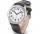The hands on the clock shows the time 12 hours and 2 minutes. Calculate the size of a sharp angle between clock hands three hours later.
• Half of halvesHalf of the square we cut off, then half of the rest, etc. Five cuts we made in this way. What part of the content of the original square is the content of the cut part?
• Summerjob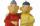Albert, Beny, Cyril and Dan worked on summerjob. From the total revenue Albert received 2/5, Beny 1/6 , 3/10 Cyril and Dan the rest which is 324 euros. How many euros earned every one of them?
• BucketThe bucket half filled with water weighs 5.55 kg, the full bucket weighs 9.85 kg. How much does the bucket weigh?
• Dividing moneyMilan divided 360 euros in the ratio 4: 5, Erik in the ratio 500: 625. How many euros did the individual parts of Milan and how many Erik?
• Nádoba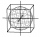Nádoba tvaru kostky je naplněna vodou do poloviny své výšky. Pokud dolijeme 20 l vody, bude nádoba naplněna do tří čtvrtin své výšky. Jaký je objem celé nádoby?
• Cyclist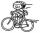The cyclist goes uphill 10 km for 50 minutes and downhill minutes for 29 minutes, both applied to the pedals the same force. How long he pass 10 km by plane?
• AgesFather is 36 years old, his daughter is 4 years old. Write down the ratio of the age of father and daughter. In what ratio will the ages of father and daughter after 4 years?
• The dealer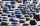The dealer planned to sell 40% of the cars he had in stock in May. However, he sold 20 less cars, ie only 15% of cars. a) How many cars did he have in stock? b) How many cars did he sell in May?
• Lookout towerCalculate the height of a lookout tower forming a shadow of 36 m if at the same time a column 2.5 m high has a shadow of 1.5 m.
• Two diggersTwo diggers should dig a ditch. If each of them worked just one-third of the time that the other digger needs, they'd dig up a 13/18 ditch together. Find the ratio of the performance of this two diggers.
• Magic bagEach time the prince crossed the bridge, the number of tolars in the magic bag doubled. But then the devil always conjured 300 tolars for him. When this happened for the third time, the prince had twice as much as he had in the beginning. How many tolars
• Stones in aquariumIn an aquarium with a length of 2 m, 1.5 m wide and 2.5 m deep, the water is up to three-quarters of the depth. Can we place 2m cubic meters of stones in the aquarium without spilling water? (0 = no, 1 = yes)
• Tape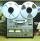Video is 153% more expensive than tape recorder. How many percent is tape recorder less expensive than video?
• Square metal sheetFour squares of 300 mm side were cut out from a square sheet metal plate with a side of 0,7 m. Express the fraction and the percentage of waste from the square metal sheet.
• Alloy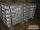The first alloy is a mixture of two metals in the ratio 1:2, the second is a mixture of same metals in the ratio 2:3. At what ratio we have these two alloys put into the furnace to obtain a new metal alloy with ratio 17:27? (All three ratios correspond to
• Mystery of stereometrieTwo regular tetrahedrons have surfaces 88 cm2 and 198 cm2. In what ratio is their volumes? Write as a fraction and as a decimal rounded to 4 decimal places.
• Divide in ratioLine segment AB 12 cm long divide in a ratio of 5: 3. How long are the individual parts?Lucy, Tereza, and Petra decided to compile the compulsory reading records together. It would take 30 days for Lucia alone, 36 for Tereza and 45 for Petra. How long will it take to make records if they will work together?
• BeerAfter three 10° beers consumed in a short time, there is 5.6 g of alcohol in 6 kg adult human blood. How much is it per mille?

Do you have an interesting mathematical word problem that you can't solve it? Submit a math problem, and we can try to solve it.

We will send a solution to your e-mail address. Solved examples are also published here. Please enter the e-mail correctly and check whether you don't have a full mailbox.

Please do not submit problems from current active competitions such as Mathematical Olympiad, correspondence seminars etc...

Need help calculate sum, simplify or multiply fractions? Try our fraction calculator.